# Linked List in Data Structures

21 September, 2018

Ratings

#### Related Blogs

The term “list” refers to a linear collection of data items. One way to store such data is by means at “array” . The array implementation of list hap certain draw backs there are

1. Memory storage space is wasted, very often the list is much stories than the array size declared.
2. List cannot grow in its size beyond the size at the declared array i.e required during paragraph execution.
3. Operations like insertion and declaration as a specified location in  a list requires a lot of movement of data, Therefore leading to inefficient and time containing algorithm
4. Another way of storing a list in memory is by means of linked list. Each element in the list contain a field, called a link fields, Which contains the address of the next elements in the list need not occupy adjacent space in memory. Thus will make it easier to insert and delete element in the list.

1. Linked list is a dynamic structure where as array is a static structure. That is the size of the array is fixed, it cannot be increased or decreased during execution of a program.
2. In portion and deletion of nodes require no data movement
3. Joining two arrays is very tedious process because these is lot of data movement, where of joining two linked list is very easy.

### Types of linked list :

These are 3 types.

• A singly linked list or one way list is a linear collection of data elements, called nodes
• Here each node is dividing into two fields.
• The first filed containing the information of the element, and the second field contains the address in the next node in the list.
• The first filed is called “Data field ” and the second field is called “Link field”• The left part represents information part of the node the right part represents the link field of the node.
• The pointer to the last node contains a special value called “NULL” pointer.
• Linked list also contains a pointer variable, called HEAD, which points to the first node of the list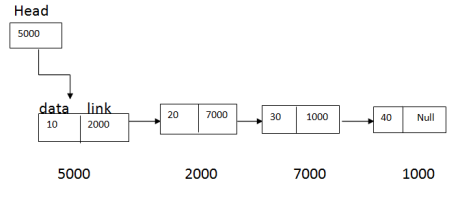### Inserting at an element in list:

1. Inserting at the beginning of the list :
• Suppose a node N is to be inserted into the list at beginning as Shan in the fig. The simplest strategy for an item at the beginning at the list ip to:
1. Allocate space for a new node.
2. Copy the item to be inserted into it.
3. Make the new nodes next pointer point to the current head of the list
4. Make the HEAD of the list point to the newly allocated node.
2. Hand = Cur;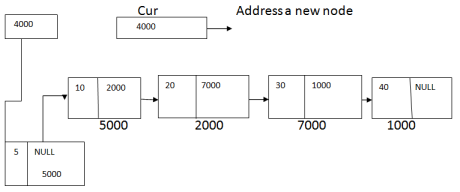### Insertion After a give node :

To insert a node in the linked list we need to specify the element to be inserted and the element in the list after which the element has to be inserted.

1. Search for the element after which the insertion is to be done.
2. Create a memory space for element to be inserted.
3. Assign the data value its data field.
4. Assign is a pointer to pointer of the element searched at step 2
5. Assign pointer of the element searched at step 2to this new element started.

2. ptr     - > Link       =cur;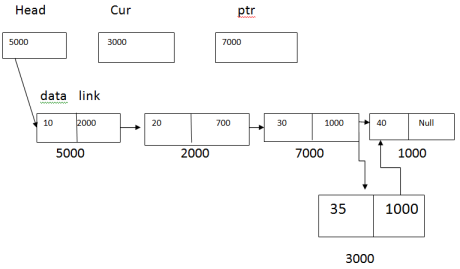### Deletion Operation :

Deleting Beginning Node:

2. Disappear the memory space held by the deleted element

Head=Head - > Link free(otr);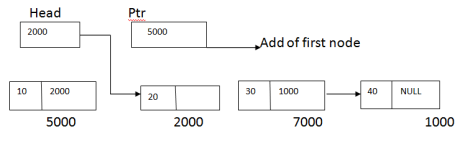### Deleting a specified node:

1. Search for the element with key value same as the element to be deleted.
2. Its pointer to next element be saved and late assigned to next element of predecessor of the element searched at step.
3. Dispose the memory space held by the deleted element.

ptr1=ptr àlink; ptr à link= ptr1 àlink; free(ptr1);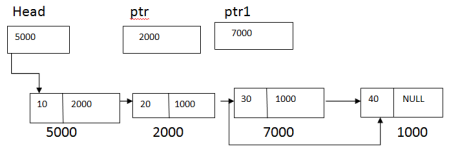Implementation of singly linked list # include <studio.h> #include <conio.h> #include<stdlib.h> struct Node { int Data; struct Node *link; }; struct Node*Head; void createList() { int else; struct Node*ptr,*cur; Head=NULL; printf(“Enter Data for the Node -1): “); scanf(“%d”,&ele); while(ele!=-1) { cur=(struct Node*)malloc (size of (structNode)); cur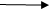Data=ele; curLink=NULL; if (Head==NULL) Head=cur; else ptrlink =cur; ptr=cur; printf(“Enter Data For The Node -1):”); scanf(“%d”,&ele); } } void Insertion() { int ele,x; char ch; struct Node*ptr,*cur; ptr=Head; cur=(structNode *) malloc (size of (structNode)); printf(“Enter Data for the New Node:”); scanf(“%d”,&x); curData=x; curLink=NULL; printf(“Do you want to insert first(y\n:”); fflush(stdin); scanf(“%c”,&ch); if((ch==’y’) || (ch==’n’)) { curlink =Head; Head =cur; } else { printf(“After which element you want to Insert:”); scanf(“%d”,&ele); while(ptr!=NULL) { if(ptrData ==ele) { curLink =ptr à Link; ptrLink= cur; break; } else { ptr =ptrLink; } } } } void Deletion () { int ele; char ch; struct Node*ptr,*ptr1; ptr=Head; printf(“Do you want to Delete First (Y/n):”); scanf(“%c”,&ch); if(ch==’y’|| ch==’n’) { Head=HeadLink; free(ptr) } else { printf(“ which element you want to delete:”); scanf(“%d”, &ele); while(ptr!=NULL) { if(ptrLink àData==ele) { ptr1=ptrLink; } } } } void Display() { struct node*ptr; ptr=Head; if(ptr==NULL) { printf(“List is Empty \n”); } else { while(ptr!=NULL) { printf(“%5d”,ptr Data); ptr=ptrLink; } } } void main() { int choice; createlist() printf(“1.INSERTION \n”); printf(“2.DELETION  \n”); printf(“3.DISPLAY  \n”); printf(“4.EXIT \n”); do { printf(“Enter your choice:”); scanf(“%d”,&choice); switch (choice) { { case1: insertion (); break; case2: deletion (); break; case3: Display(); break; case4: exit(0); } } while (choice<=4); getch(); }